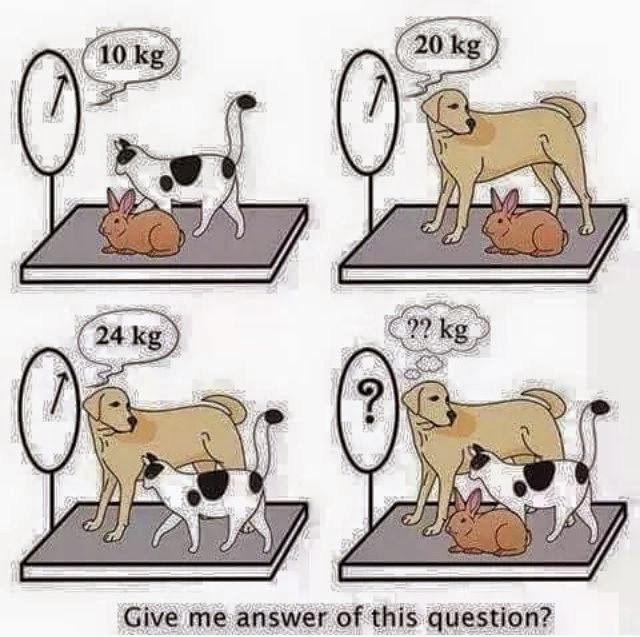# Dog Cat and Rabbit Weight Whatsapp PuzzleDog , Cat and Rabbit Puzzle
Give me answer of this question ?
Cat + Rabbit = 10 kg
Dog + Rabbit = 20 kg
Dog + Cat = 24 kg
Dog + Cat + Rabbit  = ?? kg

Answer with Explanation :
Lets say rabbit, cat and dog’s weight are R,C and D respectively.
Then by the given data :
Equation 1: R + C = 10
Equation 2: D + R = 20
Equation 3: C + D = 24
Now we need to find the value of C + R + D
On adding Equation 1,2 and 3
2R + 2C + 2D = 54
R + C + D = 27
Hence Weight of Rabbit + Cat + Dog = 27 kg